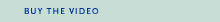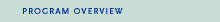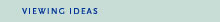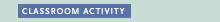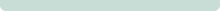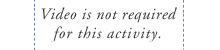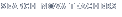Back to Teachers HomeWar Machines of TomorrowClassroom ActivityObjective
To simulate the Global Positioning System by using triangulation to locate a target.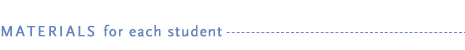• copy of "The Third Side" student handout (HTML)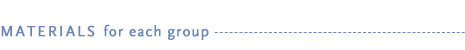• A piece of string several meters long that will stretch from ceiling to floor from an angle

• A tape measure
• graph paper
• pencils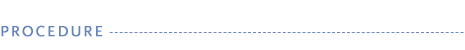1. Some rocket guidance systems use a geometric process known as triangulation. The simplest type of triangulation involves a right triangle whose sides are labeled A, B, and C, with the hypotenuse, or longest side, labeled as C. By replacing the letter variables with the known lengths of two sides, the Pythagorean theorem, A2+ B2 = C2, can be used to calculate the length of the third side.

2. The Global Positioning System features satellites that transmit their locations, thus allowing a missile to coordinate its position and plot its path to its target.

3. Students can use this activity to perform a group triangulation activity. Divide the class into groups of two or three students. Gather materials for each group and give each student a "The Third Side" student handout. Tell students to follow the procedure step-by-step.

4. After completing the activity, review the similarities and differences between the activity and an actual "smart" bomb using the GPS to strike its target.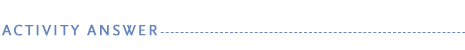Calculating the length of the third side of the triangle is a straightforward algebraic exercise. Some students may have difficulty visualizing the triangle that they are measuring or determining which side of the triangle is the hypotenuse. Encourage all students to sketch the triangle on graph paper with a pencil, and to adjust the lengths of each side as they take their measurements. Calculating square roots can be another challenge. If calculators are available, they may be helpful. As they discuss the similarities and differences, students may note that a difference between the GPS system and the activity is the GPS system involves more than just simple triangulation. With the GPS, a rocket trajectory needs to take into account the earth's curvature and weather conditions which affect the ability to accurately locate and hit targets.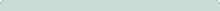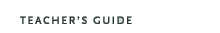War Machines of Tomorrow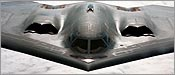Original broadcast:February 20, 1996